Based on the ecma-262 language specification, the mandatory data type conversion rules of JS are interpreted

Zheng Yudong 2021-09-15 09:40:12

Preface

js Medium Data type conversion , It has always been a piece of knowledge difficult to master in this language , Because there are many and miscellaneous conversion rules , It's easy to mix and forget .

Knowledge of this feature , The most recommended learning material is to look directly at ECMAScript Standard documents , Because it records the most authoritative and accurate js Rule of grammar , This paper can be regarded as an interpretation of this specification , It is recommended to learn from the original document .

MDN Such information websites or some js Grammar books are designed to help readers understand knowledge points faster , Will intentionally or unintentionally simplify the knowledge structure , This often leads to the loss or mistake of some knowledge , And it often carries the author's very subjective understanding （ Private goods ）, For example, in many countries js In books Implicit cast Said to be dangerous 、 Obscure and bad design , This leads me to see that many company level projects use eslint The way , No use ==. indeed , This is very meaningful in ensuring that the team's code style is unified , Because from a team perspective , It cannot be assumed that everyone is right == The conversion rules are very clear , So a one size fits all approach , It can avoid abusing such grammar , But in actual project development , Often things go against one's wishes , For example, your boss asks you to maintain a project with a lot of historical burden （ Shishan mountain ）, This syntax is widely used , Then you can only complain （ run ） outside , What we should do more is to face the difficulties , understand js The whole picture of the language , For example, in this article Type conversion rules , Only in this way can we have a clear idea , Be prepared against want .

Text

First ,js Cast in , Finally, the basic type value is always returned , So you've never heard of , A string is cast to an object .
js Cast in , Can be divided into Explicit cast （ It happened in Runtime ） And Implicit cast （ It happened in Compile time ）.

let a = 1
let b = a + "2"; // Implicit cast
let c = String(a) + "2"; // Explicit cast
Copy code

Conversion rules

ES5 Regulation No 9 In the festival Defined some “ Abstract operations ” The rules , Here we focus on ToStringToNumber and ToBoolean,ToPrimitive.

ToPrimitive

It is responsible for handling object types to Basic types Forced conversion of .ES5 standard 9.1
Object to basic type is a common operation in code , The specific implementation is through DefaultValue Operation execution conversion rule , The specific steps are ：

1. Check whether the object has valueOf() Method . Some words , Just call and execute this method , See if the return is a basic type value , If all of these are satisfied , Use this value for cast , Not satisfied, go on to the next step .
2. Check whether the object has toString() Method . Some words , Just call toString(), If there is a return value , Just use this value to cast .

If valueOf() and toString() Do not return the basic type value , Will throw out TypeError error . It's worth noting that , Use Object.create(null) Objects created [[Prototype]] The attribute is null, No inheritance valueOf() and toString() Method , Cannot cast .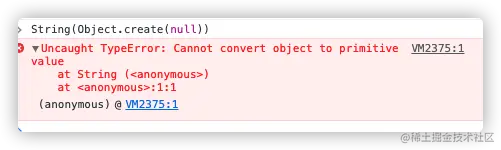ToString

It is responsible for handling non string types to String type Cast of . ES5 standard 9.8 .

1. For basic type values
It's basically what the literal length looks like , Just turn the string what it looks like .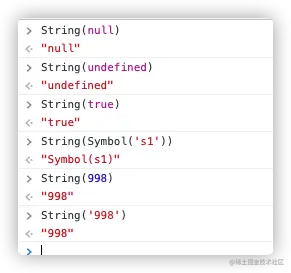1. Value for object type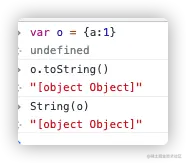Object to string , follow ToPrimitive standard , First try to convert the object to a basic type value , Then cast .

const obj = {};
obj.valueOf({}) // Return to oneself , Still object type , Non basic type , Keep going toString
obj.toString() // return “[object Object]", Is the basic type value , And the type is string , So the conversion result is it
Copy code

I wrote before JS Data type detection... Those things It is mentioned in this article that ,Object.prototype In the top object of the prototype chain , Defined valueOf,toString Method , These two methods will be inherited by all instance objects , But as mentioned earlier ToPrimitive The specification only stipulates to find these two methods from the object instance itself , Not like this Object.prototype.toString.call(xx) Directly call the corresponding method on the object at the top of the prototype chain , So on an instance, if you redefine these methods with the same name , I can modify it ToPrimitive The results of the , in fact ,js The common built-in object types in have basically realized their own exclusive functions toString Method , Therefore, the following test situations will occur .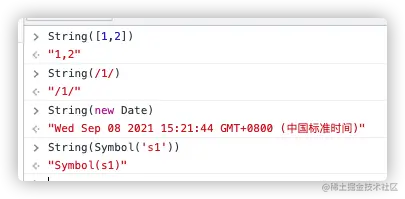Array.prototype.toString.call([1,2,3]) // "1,2,3"
Object.prototype.toString.call([1,2,3]) // "[object Array]"
Array.prototype.toString === Object.prototype.toString // false
Copy code

Code above , It is confirmed that the array is not used Object.prototype How to prototype objects , It's about realizing your own toString Method , String elements are concatenated with commas and returned , Regular returns a literal representation string , The date will return a string time stamp .

ToNumber

It handles non numeric types to Numeric type Cast of .ES5 standard 9.3.1 The method of conversion is generally to call Number(x)+ xx * 1 etc. , The second is because it is the most concise , So the most popular .

1. Base type value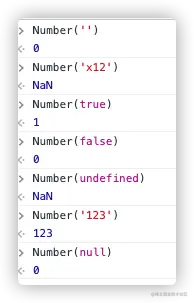among falsenull'' Convert to 0, true Convert to 1,undefined Convert to NaN . String to number , Follow the syntax of numbers , If it contains characters that are not numbers, the conversion will fail , return NaN.

1. Object type value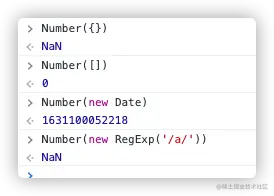Most object types to numeric types are NaN, But in the test above Array And date It's an exception , It will be converted to numbers normally . Let's press ToPrimitive The rules analyze how common object types are converted ？

const obj = {a:1};
obj.valueOf();// Returns its own object , Non basic type values , Keep going toString
const strTag = obj.toString();// return "[object Object]", Is the basic type , It's a string type , Non numeric type , So perform the following cast
Number(strTag) // NaN String to “[object Object]” perform ToNumber transformation , The final result is NaN
Copy code

Then why on the array , It will be different ？

const arr = [];
arr.valueOf(); // Return to oneself , Non basic type values , Keep going toString
arr.toString(); // return ‘’ Empty string , Base type value ,
Number(''); // Empty string ‘’ What's the number 0
Copy code

The empty array is like this , There are elements in the array

const arr = ;
arr.valueOf(); // Return to oneself , Non basic type values , Keep going toString
const str = arr.toString(); // return ‘1’, Base type value
Number(‘1’); // What's the number 1
Copy code

But not with multiple elements , The result is NaN 了

const arr = [1,2];
arr.valueOf(); // Return to oneself , Non basic type values , Keep going toString
const str = arr.toString(); // return ‘1,2’, Base type value
Number(‘1,2’); // String to number because there is an illegal comma in the middle , So in the end NaN
Copy code

This is also a classic interview question Why are empty arrays converted to numbers 0, Next time you meet, you will know how to answer the interviewer ～. There is another problem left , So why does the date normally turn into a number . Because the date object is redefined valueOf() Method , The call returns a numeric timestamp , therefore , In the first ToPrimitive The first step （ Judge valueOf Return value ） After the end , Just turn it directly to the number type .

const now = new Date();
now.valueOf() // 1631102131908 The timestamp of the present time
Date.prototype.valueOf.call(now) // 1631102131908 The timestamp of the present time
Object.prototype.valueOf.call(now) // Wed Sep 08 2021 19:55:31 GMT+0800 ( China standard time )
Date.prototype.valueOf === Object.prototype.valueOf // false
Copy code

ToBoolean

It handles non Boolean types to Boolean type Cast of .ES5 standard 9.2
This is the best thing to remember ,undefined''null0NaN These are all in js It's called False value ,Boolean() It's going to be false, besides , Other values are true values , All turned into true, In other words, all objects are true values . It is worth noting that (new Boolean(false) This wrapper object type , perform Boolean(new Boolean(false)) And will return to true, But that's just callback The above mentioned ： Objects are true this sentence .

Implicit conversion in operator and condition judgment

plus +

1 + 2 // 2
1 + '2' // '12'
false + 1 // 1
Copy code

In a nutshell , If + One of the operands of is a string , Then perform string splicing ; Otherwise, add numbers .
What about types other than numbers and strings ？ Like array types

 + [2,3] // '12,3'
Copy code

Old rules , First use ToPrimitive The rules , To the base type of a number or string class ,'1' + '2,3', Both sides are strings , The final results '12,3'. For object types + No. splices , There is a pit that is often mentioned

[] + {}; // "[object Object]"
{} + []; // 0
Copy code

On the surface + The operator is based on the first operand （[] or {}） Different will produce different results , It seems to follow + The left and right writing order of the number is related to . But it is not , In the first line of code ,{} Appear in the + Operator expression , So it is treated as a value （ An empty object ） To deal with it . I said before [] Will be cast to "", and {} Will be cast to "[object Object]". But in the second line of code ,{} As a separate empty block of code （ Do nothing ）. There is no semicolon at the end of the code block , So there is no grammatical problem here . The final code execution is actually only the latter + [] , take [] Cast type to 0... See light suddenly .jpg

Take the opposite !

As mentioned earlier + similar , Unary operator ! Explicitly cast values of other types to Boolean values , It also reverses the true value to the false value （ Or reverse the false value to the true value ）. So the most common way to explicitly cast a type to a Boolean value is !!, Because the second one ! Will reverse the result again . stay if(..).. In such a Boolean context , If not used Boolean(..) and !!, It will automatically and implicitly ToBoolean transformation . It is recommended to use Boolean(..) and !! To make the code clearer and easier to read .

|| and &&

js Medium || and && Different from other languages , They don't end up returning Booleans , But the value of one of the two operands . But in the process of judgment , It happened ToBoolean Implicit cast of .

let a = 42;
let b = null;
let c = "foo";
if (a && (b || c)) {
console.log( "yep" );
}
Copy code

here a && (b || c) The result is actually "foo" Instead of true, And then by if take foo Cast type to Boolean value , So the end result is true.

Loose is equal == vs Strictly ===

== Allow cast in equality comparison , and === Don't allow .ES5 standard 11.9.3

== Implicit cast occurs when comparing two values of different types , One or both of them will be converted to the same type and then compared . Compare x == y, among x and y Is the value , produce true or  false. The comparison is as follows ：

1. If the of two values The same type , Just compare whether they are equal .
for example ,42 be equal to 42,"abc" be equal to "abc". There are several unconventional situations to note .NaN It's not equal to NaN, +0 be equal to -0. Finally, the object is defined （ Including functions and arrays ） The looseness of ==, Two objects are considered equal when they point to the same reference address , Cast does not occur .
2. character string and Numbers An equal comparison between
• If Type(x) It's the number. ,Type(y) Is string , Then return to x == ToNumber(y) Result .
• If Type(x) Is string ,Type(y) It's the number. , Then return to ToNumber(x) == y Result .
let a = 42;
let b = "42";
a === b; // false
a == b; // true
Copy code
Conclusion ： Comparison of strings and numbers , The string is converted to a number and then compared .
1. Other types and Boolean type An equal comparison between
== One of the most error prone places is true and false Equality comparison with other types .
let x = true;
let y = "42";
x == y; // false
Copy code
"42" It's a truth value , Why? == The result is not true Well ？ standard 11.9.3.6-7 That's what it says ：
• If Type(x) It's the boolean type , Then return to ToNumber(x) == y Result ;

• If Type(y) It's the boolean type , Then return to x == ToNumber(y) Result .

Analyze the example carefully , First ：Type(x) Boolean value , therefore ToNumber(x) take true Cast type to 1, become 1 == "42", The two types are still different ,"42" Cast to according to the rule 42, Finally become 1 == 42, The result is false.
Let's do another example

if（[] == true）{alert('haha')}` alert Statement will not execute , because left [] ToPrimitive Convert to ‘’,ToNumber(） by 0 , Not equal to right true Converted to 1.
Copy code

This is also == The strangest place ,== Boolean values on both sides are cast to numbers .

1. null and undefined An equal comparison between
• If x by null,y by undefined, The result is true.
• If x by undefined,y by null, The result is true. That is to say, in == in null and undefined It is one thing , You can implicitly cast each other .
There's one more thing to note ,nullundefined stay == When judging , In addition to themselves, there are each other , Not equal to any value ～
null == ''// false
null == null // Certainly true
null == undefined // true
Copy code
1. Equality comparison between object and basic type value
• If Type(x) It's a string or a number ,Type(y) It's the object , Then return to x == ToPrimitive(y) Result ;

• If Type(x) It's the object ,Type(y) It's a string or a number , Then return to ToPromitive(x) == y Result .
The reason why the basic type value of this rule does not include Boolean types , I just talked about it alone , It's an exception , Any type of value ( Include object types ) With Boolean types == Compare , It is actually compared with the number transformed by Boolean value .

== The rules are basically finished , Now you can test the use cases below , Know your mastery

"0" == null; // false
"0" == undefined; // false
"0" == false; // true -- dizzy ！
"0" == NaN; // false
"0" == 0; // true
"0" == ""; // false
false == null; // false
false == undefined; // false
false == NaN; // false
false == 0; // true -- dizzy ！
false == ""; // true -- dizzy ！
false == []; // true -- dizzy ！
false == {}; // false
"" == null; // false
"" == undefined; // false
"" == NaN; // false
"" == 0; // true -- dizzy ！
"" == []; // true -- dizzy ！
"" == {}; // false
0 == null; // false
0 == undefined; // false
0 == NaN; // false
0 == []; // true -- dizzy ！
0 == {}; // false
```
Copy code

What about? , You're useless ？ And more dizzy

[] == ![] // true
Copy code

This is also a common interview question , Before judgment , First perform the... On the right operation , take [] Convert to Boolean , At the same time, reverse , According to the above ToBoolean The rules , Any object converted to boolean type is true, therefore ![] = false, According to the above false The special rules of , Before judgment , Turn to numbers first 0, Next , Left side [] perform ToPrimitive operation , Return to empty string , Compare strings with numbers , By rule , Convert an empty string to a number 0, Now both the left and right of the equal sign are numbers 0, therefore , The outcome is equal .
If you really can't remember so much == The rules , So there is also a simplification technique .

• null And undefined Like a couple , Firm and ambiguous , Only equal to themselves and each other .
• Compare two different types of values , If there is an object in it , Let's turn it to the basic value first , Then see if the types on both sides are consistent at the moment , atypism , Just turn it into a number and compare it .

If you have misjudged use cases , You can read the above rules again , All change is the same , Analyze , It will come to light . Finally, I'll give you an interview question and solution .

Implement a function , The calculation results can meet the following expected results ：